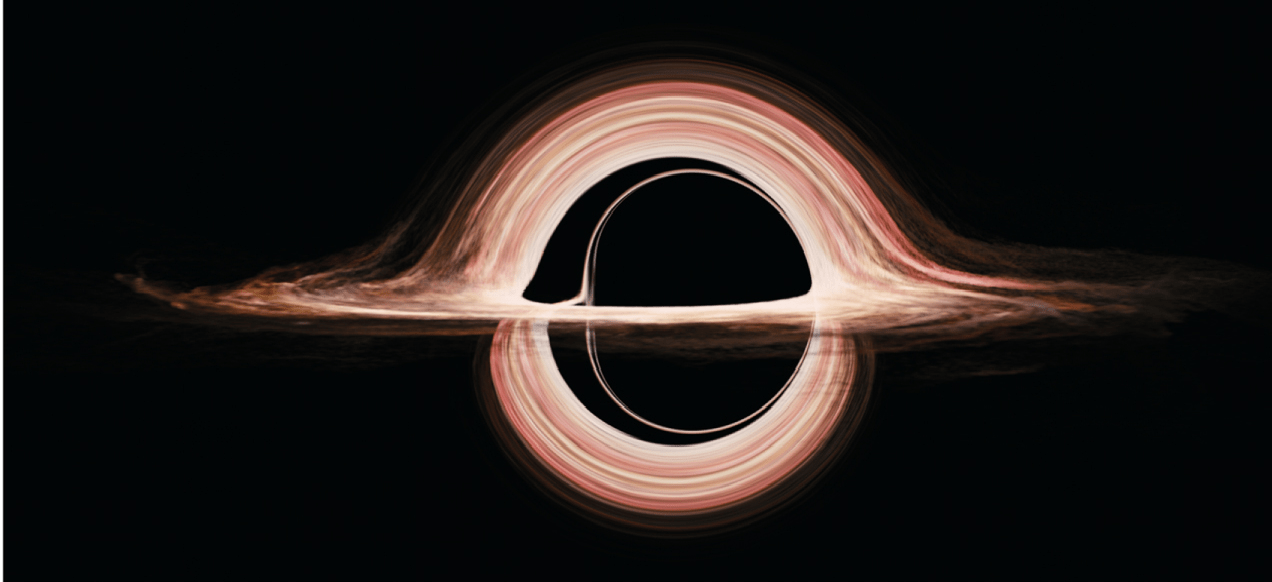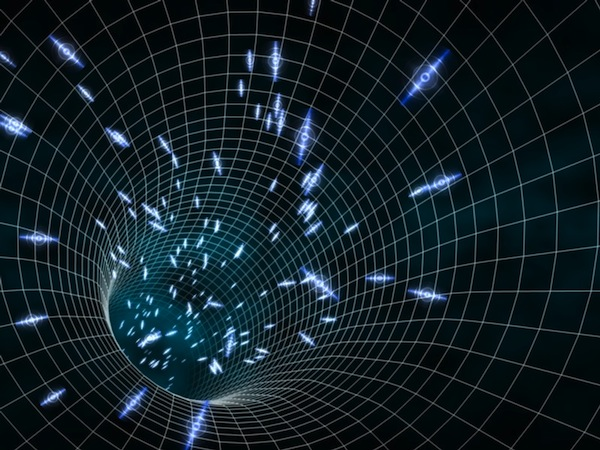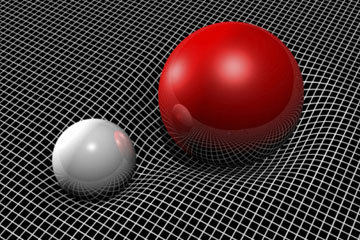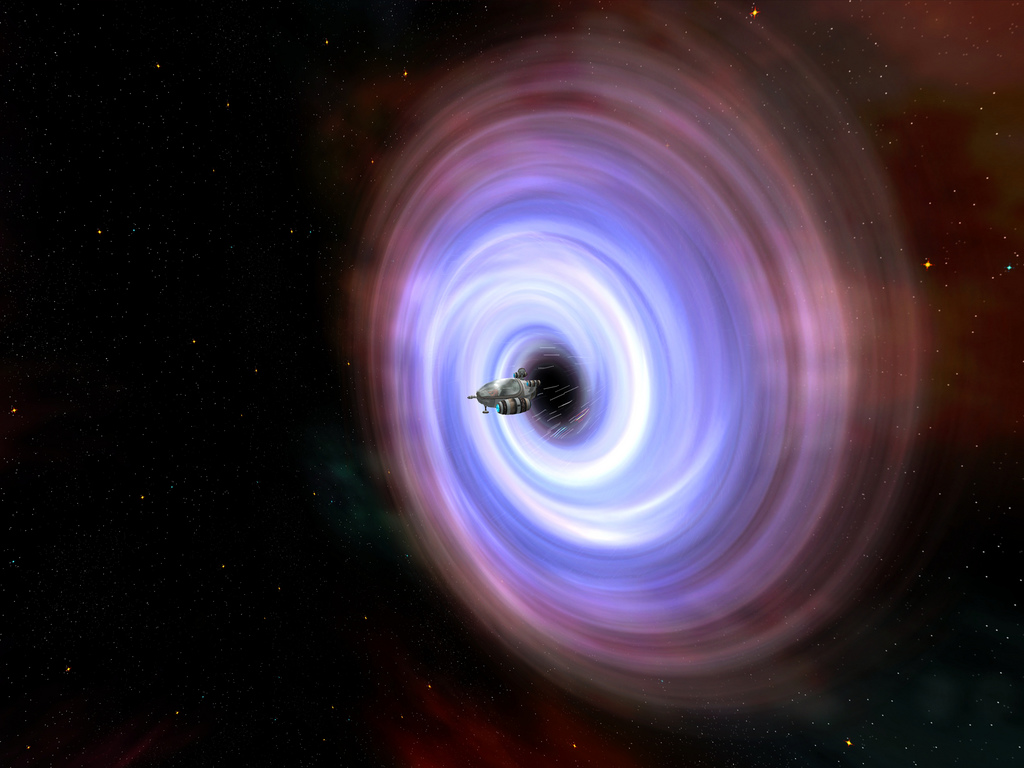# Interstellar travel: the mathematics of wormholes

Matthew Wright looks at wormholes in sci-fiWormholes have been fascinating science fiction writers for decades, allowing protagonists to travel instantaneously to remote parts of the cosmos, the distant future or even entirely different universes. Last year Christopher Nolan’s blockbuster film Interstellar featured a wormhole as its key plot device, and recently won an Oscar for its visual depiction of them. The film centres around a crew of astronauts travelling through a rip in space and time in the hope of finding a future home for humanity.Hold on tight

Although the idea of a wormhole might sound like one of the more farfetched ideas coming from the minds of science fiction writers, in fact there is a considerable amount of active research into the science behind them. The original screenplay for Interstellar was actually developed by a physicist at Caltech named Kip Thorne, who has been studying the mathematical properties of wormholes for nearly thirty years. Thorne collaborated with Double Negative Ltd, a special effects company based in Great Portland Street in central London, to ensure that the wormholes displayed in the film obeyed the correct laws of physics. And amazingly the cutting-edge super-accurate visualization software available to the Hollywood special-effects team actually enabled physicists to see new phenomena that they hadn’t anticipated.

So what are wormholes, and how can we describe them mathematically? The answer to this question requires us to know a little bit about physicists’ currently favoured description of gravity, the general theory of relativity. Kip Thorne has been one of the big names in this field of general relativity for over half a century, and so before we explore the mathematics of wormholes we will take a short detour through Einstein’s crowning achievement.

### A Very Brief Introduction to General Relativity

Albert Einstein first formulated his general theory of relativity to describe the force of gravity one hundred years ago this year (1915) and the theory has remarkably remained unchanged ever since. Einstein rejected the classical Newtonian view that gravity is just a force between massive objects. Instead, the force of gravity manifests itself by curving both space and time. What does this mean precisely? You may remember Newton’s first law, that if no force acts on an object it will either remain stationary or travel with a constant speed along a straight line. However as soon as you apply a force to an object, it will begin to accelerate and will no longer necessarily travel along a straight path.A great circle on the surface of a sphere (Source: Wikimedia Commons)

Einstein’s insight was that instead of treating gravity as a force in this regard, he could modify what it means for a line to be straight. In spaces with curvature, particles don’t move along classical Euclidean straight lines, they travel along what are called geodesics. Think of the surface of a sphere, like the earth. The quickest way to get between two points on this surface are the arcs of great circles, like the lines of longitude. These are the geodesics in this space. In Einstein’s general relativity, space and time are curved, and freely falling particles under the influence of gravity travel along the geodesics (“straight lines”) of the spacetime.

So how is this curved spacetime described mathematically? We start with a special type of vector space, called a manifold, with four dimensions: one dimension for time and three dimensions for space. On this manifold, we add some additional structure in the form of a metric $g_{ab}$ that tells us how to calculate distances and angles. The space we are all used to is traditional Euclidean flat space in Cartesian coordinates. The metric $g_{ab}$ in this case is just the identity matrix, which tells us that the line element is

$$\d s^2=\d x^2+\d y^2+\d z^2.$$

This line element allows us to calculate the length of curves in space. Einstein’s great insight was that matter distorts and curves this flat metric, and this relationship is described by the famous Einstein’s field equations,

$$R_{ab} – \frac{1}{2}R g_{ab} =\frac{8\pi G}{c^4}T_{ab}.$$Two masses curving spacetime.

On the left hand side of the equation we have terms involving the Ricci tensor $R_{ab}$ and its trace, the Ricci scalar $R$. These tensors are functions of the metric which measure the amount of curvature present at each point of our spacetime. On the right hand side we have $G$, Newton’s gravitational constant; $c$, the speed of light; and $T_{ab}$ which is called the energy momentum tensor. This describes the amount, type and distribution of energy and matter in space.

Given an energy momentum tensor describing a matter distribution, the aim is to then solve Einstein’s equation for the metric $g$. In general this is very difficult to do since the equations are highly non-linear. This is because the matter is telling spacetime how to curve, but this curvature then effects how the matter in our spacetime is moving, so we get lots of complicated feedback effects. In all but highly idealised symmetric situations finding exact solutions to Einstein’s equations is almost impossible.

### The Mathematics of WormholesModel of a two dimensional wormhole.

So how do we describe a wormhole using general relativity? Essentially a wormhole is a topological feature of our manifold connecting two completely separate regions of space with a tunnel. Topologists would call a wormhole “a handle” of a multiply-connected space. To visualise this take a flat sheet of paper and fold it in half smoothly (without creasing the fold!), cut a small hole in each half, and then connect a tube or cylinder between the two separate holes. You now have a wormhole in two dimensional space. It’s as simple as that!

Wormholes were first studied mathematically in relativity as early as 1921 by the German mathematician Herman Weyl. However it wasn’t until Thorne began studying them in the 1980s that they started to be taken seriously by relativists. Thorne’s friend Carl Sagan, one of the great popularisers of science of the twentieth century, was working on a science fiction novel Contact, which was later turned into a Hollywood movie. Sagan wanted to feature wormholes in the novel, so asked his friend Kip Thorne to look at wormhole solutions to Einstein’s equations. Shortly after, Thorne proposed the idea of a traversable wormhole that would allow an explorer to travel through a wormhole in both directions from one part of the universe to another very quickly. A whole new area of physics research was spawned.

Recall that if we are given a distribution of matter, Einstein’s equation allows us to solve for the metric of our spacetime, although we’ve already seen that this is typically a very difficult procedure. In Thorne’s 1988 paper, Wormholes in spacetime and their use for interstellar travel, he turned this procedure on its head. Instead of inputting matter into the equation and solving for the metric, he inserted the metric describing a wormhole into the left hand side of Einstein’s equation, and then derived conditions for the matter that would be required to create this wormhole. This is a much simpler procedure since we no longer need to solve a complicated set of coupled partial differential equations.

So what does the metric of a wormhole look like? Thorne and his graduate student Mike Morris proposed what is now called the Morris-Thorne metric, which is given in spherical polar coordinates by

$$\d s^2 = -e^{\Phi(r)}\,\d t^2 +\frac{1}{1-\frac{b(r)}{r}}\,\d r^2 + r^2(\d \theta^2+\sin^2\theta \,\d\phi^2).$$A Morris-Thorne wormhole. (Source: Wikimedia Commons)

This metric describes two separate regions of universe, connected by a tube. These different regions can either be interpreted as two separate universes, or the same universe at a different place in time and space. The function $b(r)$ describes the shape of the wormhole, and the throat of the wormhole, where the two different parts of the universe are connected, is located at $r_0$ where $b(r_0)=r_0$. This function has to obey a condition called the flare out condition, $b'(r)<1$, which ensures that the throat remains open.

### Exotic Matter

Relativists usually assume based on physical grounds that all matter in our spacetime obeys certain energy conditions. The weakest of these energy conditions is called the “null energy condition”. In the context of a fluid, this simply states that the pressure $p$ added to the energy density $\rho$ of the fluid at each point is greater than zero,

$$\rho+ p \geq 0.$$

This is a very sensible and physical thing to assume, classically both energy and pressure are positive for all known types of matter currently known to physicists in the universe.

However, when inserting the above Morris-Thorne metric into Einstein’s equations a problem arises. It is found that the energy required to keep the throat of the wormhole open long enough for an observer to pass through, without exceeding the speed of light, would require a violation of the null energy condition. This is because in order to keep the throat open, a repulsive gravitational force is required whereas typically gravity behaves as an attractive force.

So does that mean wormholes have to remain the fantasies of science fiction writers? Well, not necessarily! Physicists call matter that violates the null energy condition “exotic matter”. In fact, it is entirely mathematically consistent that particles with negative mass could exist, and these would violate the null energy condition. And physicists are far from knowing all types of matter in the universe. Dark energy and dark matter make up about 96% of the energy content of the universe, and we are still pretty clueless as to what this matter and energy is made of. Dark energy, which is responsible for the universe’s expansion rate accelerating, exhibits a negative pressure and in some cosmologists’ models violates the null energy condition. This repulsive pressure could be utilised to keep a wormhole open.Artistic impression from an observer’s perspective as they cross the event horizon of a wormhole. (Source: Wikimedia Commons)

Another possible saviour for wormholes comes from modified theories of gravity. It is strongly believed by many physicists that general relativity is not part of the final theory of everything that they have been searching for for centuries. The two fundamental theories of physics, general relativity and quantum field theory, are fundamentally incompatible, and many believe that general relativity will have to be modified on small scales to take account of quantum mechanics. Many also believe general relativity needs to be modified on large cosmological scales as well: current observations show that the universe is accelerating in its expansion, which cannot be explained in a fully satisfactory way without modifying relativity. Physicists have proposed many alternative theories to general relativity, and in many of these theories it is possible to get wormhole solutions to the governing equations without invoking any exotic matter.

It’s not currently believed that wormholes can exist naturally in the universe, except possibly at quantum scales, but it may be possible that a highly advanced civilisation with enough technology could build one. This would have some interesting consequences. For example, this may allow the possibility of time travel, giving rise to such paradoxes as the famous grandfather paradox: if you go back in time and kill your grandfather, how were you alive in order to go back in time and kill your grandfather? Such paradoxes might be unpalatable, and we may need new physical principles to rule out their existence. Thorne has suggested one resolution to this paradox by hypothesising that we may only be able to build wormholes that travel into the future and not the past, but it is still unclear whether this avoids all paradoxes.

Wormholes may still be incredibly speculative objects, and the technology required to build one is far beyond anything human civilisation would be capable of for many millennia. But studying them has been a very fruitful area of research for physicists exploring the mathematical properties of general relativity. Pushing a theory to its limit and looking at extreme examples allows us to explore where a theory might break down. Usually the ideas of science fiction are inspired by cutting-edge physics; this is a fascinating area where science fiction has inspired a whole new exciting field of cutting edge research.Matt is a PhD student at UCL, working in the fields of general relativity and cosmology.
ucl.ac.uk/~ucahawr    + More articles by Matthew